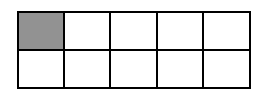### Home > CC3 > Chapter 1 > Lesson 1.1.1 > Problem1-5

1-5.

In this course, you will need to be able to work with numbers, words, and geometric representations. Use each of these representations to answer the following problems. Homework Help ✎

1. Draw and shade a figure that represents $100\%$. Label your figure “$100\%$.” Then describe the figure in words.

If you have $100\%$ of one pie, how many pies do you have?

Diagrams vary, but can be described as a whole.

1. Similarly, draw and shade figures that represent $50\%$, $25\%$, and $150\%$. Label each figure and describe it in words.

Remember that percentages are just fractions with a denominator of $100$.

Diagrams vary. One half, one fourth, one and one half.

2. Write another fraction that is equivalent to $\frac{4}{5}$. Draw diagrams to show that they are equal. Then find the equivalent decimals for both fractions and write the equivalent percent.

Remember that fractions with denominators of $100$ can be easily changed to percentages and that percentages can easily be changed to decimals.

$\frac{8}{10} \text{ etc}., 0.8, 80\%$

3. Draw and shade a figure that represents “one third.” How can this figure be represented with a number?

You have a three-piece pie and decided to eat one of the pieces. How much of the pie did you eat?

$\frac{1}{3}$

4. Describe what the diagram below represents using words and numbers.There are $10$ blocks total, but only $1$ shaded block.

One tenth, $10\%$